Next: Some advice on how Up: Conclusions Previous: About the code   Contents

Subsections

# Improvements

## Unconstrained case

The algorithm is still limited to search space of dimension lower than 50 (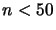). This limitation has two origin:
• The number of evaluation of the function needed to construct the first interpolation polynomial is very high: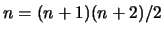). It is possible to build a quadratic using less point (using a Least frobenius norm updating of quadratic models that satisfy interpolation conditions''), as in the DFO algorithm, but the technique seems currently numerically very instable. Some recent works of Powell about this subject suggest that maybe a solution will soon be found (see [Pow02]).
• The algorithm to update the Lagrange polynomial (when we replace one interpolation point by another) is very slow. Its complexity is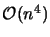. Since the calculation involved are very simple, it should be possible to parallelize this process. Another solution would be to use Multivariate Newton polynomials'' instead of Multivariate Lagrange Polynomials''.
Other improvements are possible:
• Improve the warm-start'' capabilities of the algorithm.
• Use a better strategy for the parallel case (see end of section 7.3)
• Currently the trust region is a simple ball: this is linked to the L2-norm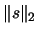used in the trust region step computation of Chapter 4. It would be interesting to have a trust region which reflects the underlying geometry of the model and not give undeserved weight to certain directions (like by using a H-norm: see Section 12.4 about this norm). The Dennis-Moré algorithm can be easily modified to use the H-norm. This improvement will have a small effect provided the variables have already been correctly normalized (see section 12.3 about normalization).
• The Dennis-Moré algorithm of of Chapter 4 requires many successive Cholesky factorization of the matrix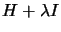, with different value of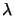. It's possible to partially transformin tri-diagonal form to speed-up the successive Cholesky factorizations, as explained in [Pow97].
• Another possible improvement would be to use a more clever algorithm for the update of the two trust regions radius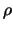and. In particular, the update ofis actually not linked at all to the success of the polynomial interpolation. It can be improved.
• Some researches can also be made in the field of kriging models (see [BDF$^+$98]). These models need very few model improvement steps'' to obtain a good validity. The validity of the approximation can also be easily checked. On the contrary, in optimization algorithms based on other models (or surrogate: see [KLT97,BDF$^+$99]) like Neural Networks, Fuzzy Set, Lazy learning, ... the validity of the model is hard to assess (there is often no mathematical tool to allow this). The surrogate approach is a serious, correct and strong theory. Unfortunately, most optimizers based on NN, Fuzzy set,... do not implement completely the surrogate approach. In particular, most of the time, these kind of optimizers doesn't care for the validity of their model. They should thus be proscribed because they can easily fail to converge, even to a simple local optimum. Furthermore, they usually need many model improvement step'' to ensure validity and turn to be very slow.

## Constrained case(12.1)
The method to solve this equation is described in Chapter 5. This method does not take into account the constraints. As a result, CONDOR may ask for some evaluations of the objective function in the infeasible space. The infeasibility is never excessive (it's limited by: see equation 12.1 ) but can sometime be a problem. A major improvement is to include some appropriate techniques to have a fully feasible-only algorithm.
Sometimes the evaluation of the objective function fails. This phenomenon is usual in the field of shape design optimization by CFD code. It simply means that the CFD code has not converged. This is referred in the literature as virtual constraints'' [CGT98]. In this case, a simple strategy is to reduce the Trust Region radiusand continue normally the optimization process. This strategy has been implemented and tested on some small examples and shows good results. However, It is still in development and tuning phase. It is the subject of current, ongoing research.